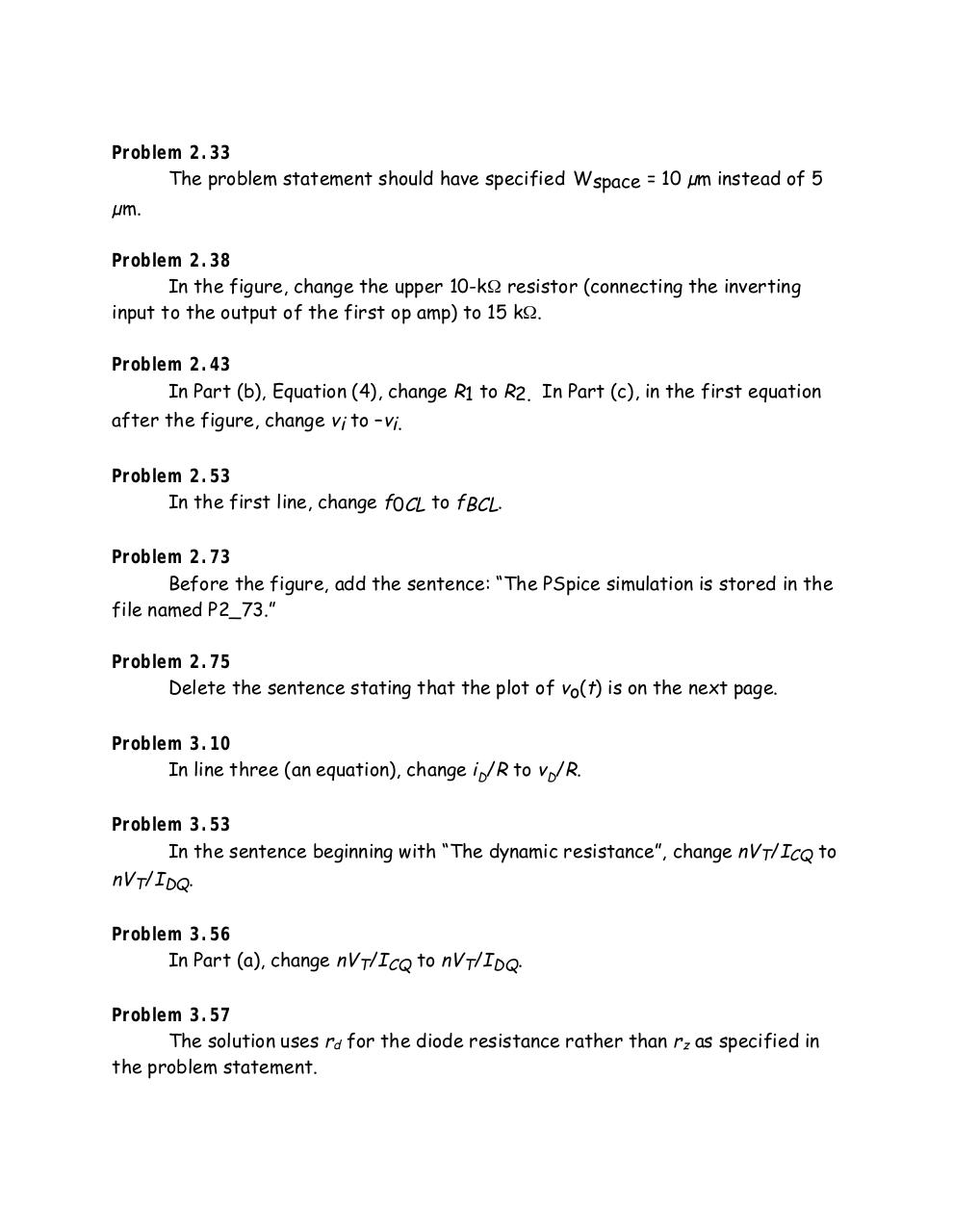# Electronics Hambley 2 edicion.pdfPage 1 234561

#### Text preview

Problem 2.33
The problem statement should have specified Wspace = 10 µm instead of 5
µm.
Problem 2.38
In the figure, change the upper 10-kΩ resistor (connecting the inverting
input to the output of the first op amp) to 15 kΩ.
Problem 2.43
In Part (b), Equation (4), change R1 to R2. In Part (c), in the first equation
after the figure, change vi to –vi.
Problem 2.53
In the first line, change f0CL to fBCL.
Problem 2.73
Before the figure, add the sentence: “The PSpice simulation is stored in the
file named P2_73.”
Problem 2.75
Delete the sentence stating that the plot of vo(t) is on the next page.
Problem 3.10
In line three (an equation), change iD/R to vD/R.
Problem 3.53
In the sentence beginning with “The dynamic resistance”, change nVT/ICQ to

nVT/IDQ.

Problem 3.56
In Part (a), change nVT/ICQ to nVT/IDQ.
Problem 3.57
The solution uses rd for the diode resistance rather than rz as specified in
the problem statement.